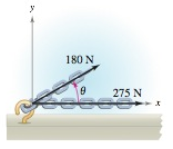Chapter 11.1, Problem 76E

Chapter
Section
Textbook Problem

# Numerical and Graphical Analysis Forces with magnitudes of 180 newtons and 275 newtons act on a hook (see figure). The angle between the two forces is θ degrees.(a) When θ = 30 ° , find the direction and magnitude of the resultant force.(b) Write the magnitude M and direction α of the resultant force as functions of θ, where 0 ° ≤ θ ≤ 180 ° .(c) Use a graphing utility to complete the table. θ 0° 30° 60° 90° 120° 150° 180° M α (d) Use a graphing utility to graph the two functions M and α .(e) Explain why one of the functions decreases for increasing values of θ, whereas the other does not.(a)

To determine

The Direction and magnitude of the resultant force.

Explanation

Given: Force with magnitude of 180 newtons and 275 newton 𝜃 =30°.

Let F1 be the force of magnitude 275 newton.

F1=275,0 As it is acting along x-axis.

F2 be the force of magnitude of 180N.

F2=180 cos30 ,180sin30=18032,180×12=                                                =

(b)

To determine

The magnitude M and Direction ∝ of the resultant force as function of θ.

(c)

To determine

Complete the table

(d)

To determine

The graph of M and α.

(e)

To determine

The reason for one of the functions decreases for increasing value of α, whereas other does not.

### Still sussing out bartleby?

Check out a sample textbook solution.

See a sample solution

#### The Solution to Your Study Problems

Bartleby provides explanations to thousands of textbook problems written by our experts, many with advanced degrees!

Get Started

#### Find more solutions based on key concepts